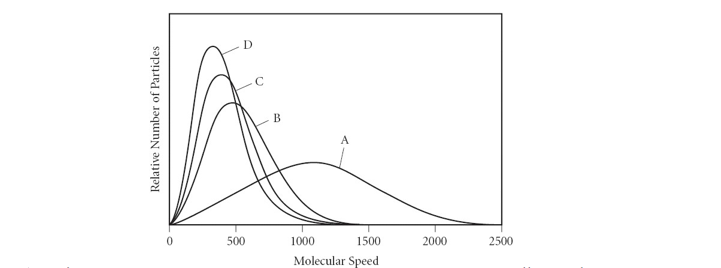# Problem: The graph (below) shows the distribution of molecular velocities.  How many of the statements are true?At a given temperature, curve A represents gas particles with the smallest molar massFor a given gas sample, curve D represents the highest temperatureAt a given temperature, curve D represents gas particles with the highest molar massFor a given gas sample, the broader the velocity distribution, the lower the temperature0                      b) 1                  c) 2                  d) 3                  e) 4

###### FREE Expert Solution

We’re being asked how many statements are true involving the distribution of molecular velocities

In the graph, the y-axis is the relative number of particles while the x-axis is the molecular speed. We can think of the molar mass of a gas as being related to the number of particles ( molar mass =  particles).###### Problem Details

The graph (below) shows the distribution of molecular velocities.  How many of the statements are true?

• At a given temperature, curve A represents gas particles with the smallest molar mass
• For a given gas sample, curve D represents the highest temperature
• At a given temperature, curve D represents gas particles with the highest molar mass
• For a given gas sample, the broader the velocity distribution, the lower the temperature
1. 0                      b) 1                  c) 2                  d) 3                  e) 4What scientific concept do you need to know in order to solve this problem?

Our tutors have indicated that to solve this problem you will need to apply the Velocity Distribution concept. You can view video lessons to learn Velocity Distribution. Or if you need more Velocity Distribution practice, you can also practice Velocity Distribution practice problems.

What is the difficulty of this problem?

Our tutors rated the difficulty ofThe graph (below) shows the distribution of molecular veloci...as medium difficulty.

How long does this problem take to solve?

Our expert Chemistry tutor, Sabrina took 3 minutes and 8 seconds to solve this problem. You can follow their steps in the video explanation above.

What professor is this problem relevant for?

Based on our data, we think this problem is relevant for Professor Bindell's class at UCF.